## NCERT  Solutions for Class 12 Micro Economics Chapter-2 Consumer Equilibrium

### NCERT TEXTBOOK QUESTIONS SOLVED

Question 1. What do you mean by the budget set of a consumer?
Or
Define Budget Set. [CBSE 2011, 13] [1 Mark]
Answer:  Budget set is the collection of all bundles of goods that a consumer can buy with his income at the prevailing market prices.

Question 2. What is Budget Line? [CBSE 2011, 13, 11C, A1 10][1 Mark]
Answer: Budget line is a graphical representation which shows all the possible combinations of the two goods that a consumer can buy with the given income and prices of commodities. It is also called consumption possibility line.

Question 3. Explain why budget line is downward sloping?
Or
Why is budget line negatively sloped? [CBSE 2011 C][l Mark]
Answer: ‘Budget line is downward sloping because if a consumer wants to buy more of one commodity, he has to buy less of other goods, given money income.

Question 4. A consumer wants to consume two goods. The prices of the two goods are Rs 4 and Rs 5 respectively. The consumer’s income is Rs 20.

1. Write down the equation of the budget line.
2. How much quantify of good 1 can the consumer consume if she spends her entire income on that good?
3.  How much of good 2 can she consume if she spends her entire income on that good?
4. What is the slope of the budget line? [3-4 Marks]

1. Let the two quantities of goods be X and Y. We are given Px = Rs 4, P = Rs 5, Consumer’s income (M) = Rs 20. Budget line equation is,
Px .X + Py .Y = M or = 4X + 5Y = 20
2. If quantity consumed of good Y = 0, Budget equation becomes,
Px.X + zero = M = 4.X = 20 = X = 20/4 = 5 units
3. If quantity consumed of good X = 0, Budget equation becomes,
Zero + Py.Y = M
or = 5Y = 20 = Y = 20/5 = 4 units.
4. Slope of budget line = Px/Py = 4/5 = 0.8

Questions 5, 6 and 7 are related to question 4,
Question 5. How does the budget line change if the consumer’s income increases to ?40 but the prices remain unchanged? [1 Mark]
Answer: If consumer’s income increases to Rs 40, the consumer can buy more pieces/quantities of both the goods X and Y. There will be parallel rightward shift in the budget line AB to A1B1.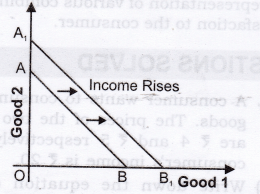Question 6. How does the budget line change if the price of good 2 decreases by a rupee but the price of good 1 and the consumer’s income remain unchanged? [ 1 Mark]
Answer: If price of good 2 (shown on y-axis) decreases, consumer can buy more pieces /quantity of good 2. The budget line AB will pivot at B and rotate upwards to A1 B.Question 7. What happens to the budget set if both the prices as well as the income double? [ 1 Mark]
Answer: There will be no change in the budget line. Let us understand this with the help of an example: Suppose,the price of goods 1 rises from Rs 4 to Rs 8 and that of goods 2 rises from Rs 5 to Rs 10. Income also rises from Rs 20 to Rs 40. With double increase in prices and income, intercepts on both X-axis and Y-axis will remain unchanged at 5 units (goods 1) and 4 units (goods 2) respectively. Slope of budget line will also remain the same. Therefore, there will be no change in the budget set and the budget line.

Question 8. Suppose a consumer can afford to buy 6 units of good 1 and 8 units of good 2 if she spends her entire income. The prices of the two goods are Rs 6 and Rs 8 respectively. How much is the consumer’s income?  [1 Mark]
Answer: Budget equation is given as: Px.X + Py .Y = M
Let good 1 be X and good 2 be Y Putting the values, we get,
(6).(6) + (8).(8) = 36 + 64 = Rs 100

Question 9. Suppose a consumer wants to consume two goods which are available only in integer units. The two goods are equally priced at Rs 10 and the consumer’s income is Rs 40.

1. Write down all the bundles that are available to the consumer.
2.  Among the bundles that are available to the consumer’s. Identify those which cost her exactly 40.[3-4 Marks]

Answer: Let Px = Py = Rs 10
Money Income = 40

1. Bundles available to consumer are: (0,0), (0,1), (0,2), (0,3), (0,4), (1,0), (1,1), (1,2), (1,3), (2,0), (2,1), (2,2), (3,0), (3,1) and (4, 0).
2.  (0,4), (1,3), (2,2), (3,1) and (4,0) cost exactly Rs 40. All the other bundles cost less than Rs 40.

Question 10. What are monotonic preferences? [AI 2011 C][l Mark]
Answer: Consumer’s preferences are assumed to be such that between any two bundles (x1, x2) and (y1, y2), if (x1, x2) has more of at least one of the good and no less of the other good as compared to (y1, y2), the consumer prefers (x1, x2) to (y1, y2). Preferences of this kind are called monotonic preferences.

Question 11. If a consumer has monotonic preferences, can she be indifferent between the bundles (10, 8) and (8, 6)? [1 Mark]
Answer: No, if a consumer has monotonic preferences, bundle (10, 8) is preferred to bundle (8, 6) as bundle (10, 8) has more units of both the goods.

Question 12.  Suppose a consumer’s preferences are monotonic. What can you say about her preference ranking over the bundles (10, 10), (10, 9), (9, 9).  [1 Mark]
Answer: If a consumer has monotonic preferences then,

1. Bundle (10, 10) is monotonically preferred to bundle (10, 9) and bundle (9, 9).
2. Bundle (10, 9) is monotonically preferred to bundle (9, 9).

Question 13. Suppose your friend is indifferent to the bundles (5, 6) and (6, 6). Are the preferences of your friend monotonic? [I Mark]
Answer: No, the preferences of my friend are not monotonic since bundle (6, 6) should be monotonically preferred to bundle (5, 6).

### MORE QUESTIONS SOLVED

I. Very Short Answer Type Questions (1 Mark)
Question 1. Define utility. [CBSE 2005C, 06C, AI 06]
Answer: Utility is the power or capacity of a commodity to satisfy human wants.

Question 2. Define total utility.
Answer: Total utility is the sum of all the utilities derived from consumption of all the units of a particular commodity.

Question 3. How much is total utility at zero level of consumption?

Question 4. How is total utility deriveds from marginal utility?
Answer: TU = MU1 + MU2 + MU3 +————- +
$$M{ U }_{ N }=\Sigma MU$$

Question 5. Define marginal utility. [AI 2006, Foreign 2006]
Answer: Marginal utility is the additional utility derived from consumption of an additional unit of a commodity.

Question 6. What is consumer’s equilibrium? [Foreign 2006, CBSE 2009C, AI 2013C]
Answer: Consumer’s equilibrium refers to a situation where a consumer gets the maximum satisfaction out of his given money income and given market price.

Question 7. What is meant by MU of one rupee?
Answer: MU of one rupee refers to the utility obtained from purchase of commodities with one rupee.

Question 8. Define indifference curve.  [AI 2013, 2014, 2015]
Answer: Indifference curve refers to the graphical representation of various combinations of the two goods that provide the same level of satisfaction to a consumer.

Question 9. Define indifference map?  [CBSE 2013C, AI 2015]
Answer: A set of indifference curves is called indifference map.

Question 10. Define marginal rate of substitution.
Answer: MRS is the rate at which a consumer is willing to give up one commodity for an extra unit of other commodity without affecting his total satisfaction.

Question 11. Why are indifference curves always convex to the origin?
Answer: Indifference curves are always convex to the origin because of the diminishing marginal rate of substitution.

Question 12. Why does an indifference curve slope downwards?
Answer: An Indifference curve slopes downwards because increase in units of one good requires decrease in the number of units of the other good to maintain the same level of satisfaction.

Question 13. Give equation of Budget Line. [CBSE 2015]
Answer: P1X1 + P2X2 = M.

Question 14. Give equation of Budget Set. [CBSE 2015]
Answer: P1X1 + P2X2 < M.

Question 15. Define Budget Set. [CBSE 2015]
Answer: It is the collection of all bundles of pieces of goods that a consumer can buy with his income at the prevailing market prices.

Question 16. Define Budget Line.  [AI 2015]
Answer: Budget line is a graphical representation which shows all the possible combinations of the two goods that a consumer can buy with the given income and prices of commodities.

II. Multiple Choice Questions (1 Mark)
Question 1. Total utility is maximum when
(a) Marginal utility is zero.
(b) Marginal utility is at its highest point.
(c) Marginal utility is equal to average utility.
(d) Average utility is maximum.

Question 2. Which of the shaded area in the diagrams below represent total utility?  [CBSE Sample Paper 2014]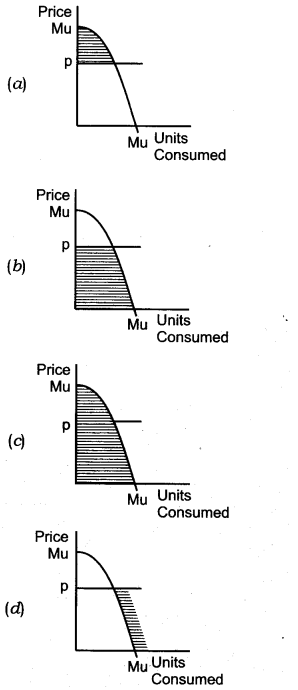Question 3. What does the area under the marginal utility curve depict? [CBSE Sample Paper 2014]
(a) Average Utility
(b) Total Utility
(c) Indifference Curve
(d) Consumer Equilibrium

Question 4. Which one of the following is not an assumption of the theory of demand based on analysis of indifference curve?
(a) Given scale of preferences as between different combinations of two goods.
(b) Diminishing marginal rate of substitution.
(c) Constant marginal utility of money.
(d) Consumers would always prefer more of a particular piece of goods to less of it, other things remaining the same.

Question 5. The consumer is in equilibrium at a point where the budget line—
(a) Is above an indifference curve.
(b) Is below an indifference curve.
(c) Is tangent to an indifference curve.
(d) Cuts an indifference curve.

Question 6. An indifference curve slopes down towards right since more of one commodity and less of another result in—
(a) Same satisfaction.
(b) Greater satisfaction.
(c) Maximum satisfaction.
(d) Decreasing expenditure.

Question 7. The second glass of lemonade gives lesser satisfaction to a thirsty boy. This is a clear case of
(a) Law of demand.
(b) Law of diminishing returns.
(c) Law of diminishing utility.
(d) Law of supply.

Question 8. The consumer is in equilibrium when the following condition is satisfied:
(a)$$\frac { M{ U }_{ x } }{ M{ U }_{ y } } >\frac { { P }_{ x } }{ { P }_{ y } }$$
(b)$$\frac { M{ U }_{ x } }{ M{ U }_{ y } } <\frac { { P }_{ x } }{ { P }_{ y } }$$
(c)$$\frac { M{ U }_{ x } }{ M{ U }_{ y } } =\frac { { P }_{ x } }{ { P }_{ y } }$$
(d)None of these.

Question 9. Which of the following options is a property of an indifference curve?
(a) It is convex to the origin.
(b) The marginal rate of substitution is constant as you move along an indifference curve.
(c) Marginal utility is constant as you move along an indifference curve.
(d) Total utility is the greatest where the 45 degrees line cuts the indifference curve.

Question 10. When economists speak of the utility of a certain good, they are referring to-
(a) The demand for the good.
(b) The usefulness of the good in consumption.
(c) The satisfaction gained from consuming the good.
(d) The rate at which consumers are willing to exchange one unit of good for an other one.

Question 11. Budget set is—
(a) Right angled triangle formed by the budget line with the axes.
(b) All points on the budget line.
(c) Points inside the budget line.
(d) Points on Y-axis from where budget line starts and the point on X-axis where budget line ends.

Question 12. If indifference curve is straight line downward sloping,
(a) MRS is increasing
(b) MRS is decreasing
(c) MRS is constant
(d) MRS is zero

Question 13. If X and Y are two commodities, indifference curve shows—
(a) X and Y are equally preferred
(b) Y is preferred to X
(c) X is preferred to Y
(d) None of these.

Question 14. If Marginal Rate of Substitution is constant throughout, the Indifference curve will be:  [CBSE 2015]
(a) Parallel to the x-axis.
(b) Downward sloping concave.
(c) Downward sloping convex.
(d) Downward sloping straight line.

Question 15. If Marginal Rate of Substitution
is increasing throughout, the Indifference curve will be:  [AI 2015]
(a) Downward sloping convex.
(b) Downward’ sloping concave.
(c) Downward sloping straight line.
(d) Upward sloping convex.

Question 16. Which of the can be referred to as ‘point of satiety’?
(a) Marginal Utility is negative
(b) Marginal utility is zero
(c) Total Utility is rising
(d) Total Utility is falling
[CBSE Sample Paper 2016]

III. Short Answer Type Questions (3-4 Marks)
Note: Questions 1, 2 and 3 have same figure.
Question 1. Explain with diagram, the relationship between TU and MU. [CBSE Foreign 2011]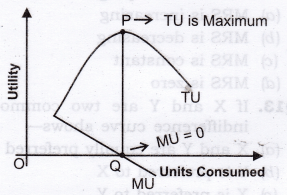1. When MU decreases, TU increases at a diminishing rate. (As shown in figure till consumption level OQ).
2. When MU is zero, TU is constant and maximum at P.
3. When MU is negative, TU starts diminishing.

Question 2. How many chocolates will a consumer have, if they are available free of cost?
Answer:  In case of free chocolates, consumer will carry on the consumption till his total utility is maximum. It means,till the additional chocolates gives positive satisfaction, consumer will keep on having chocolates. Let us understand this with the help of the figure shown in Question 1. Consumer will stop the consumption at the point of satiety (Point ‘Q’), i.e., where marginal utility is equal to zero.

Question 3. “Total Utility remains the same, whether Marginal Utility is positive or negative”. Defend or refute.
Answer:  The given statement is refuted. When Marginal Utility is positive till point Q as shown in figure of Question 1, then total Utility increases at a diminishing rate and when Marginal Utility is negative after point Q, total Utility decreases.

Question 4. State with reasons if the following statements are true or false:

1. At a grand family get-together party you go on eating and eating since you have not to pay.
2. As we consume more units of a commodity, our total utility from its consumption keeps falling.

1. False: For free goods, a consumer will limit his consumption of a commodity to a point where the point of full satisfaction is reached. Consumption beyond this point will only generate disutility.
2. False: As we consume more units of a commodity, it’s marginal utility keeps on diminishing. Total utility keeps on rising, but at a diminishing rate till marginal utility becomes zero.

Question 5. Explain the law of diminishing marginal utility with the help of a total utility schedule. [CBSE 2005C, 06C, 10, IOC, AI 2006, CBSE 13]
Answer:  The law states that marginal utility derived from the consumption of a commodity declines as more units of that commodity are consumed.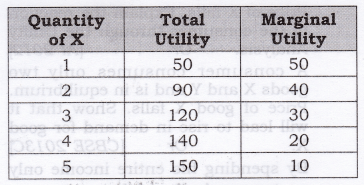It can be seen from the above schedule that total utility increases at a diminishing rate, which leads to fall in marginal utility.

Question 6. Derive MU Schedule from TU Schedule.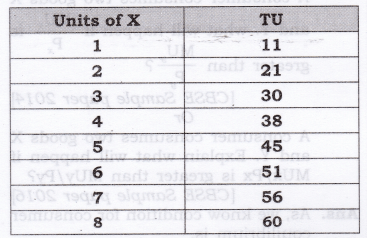Question 7.  A person’s marginal utility schedule is given below. Derive their total utility schedule.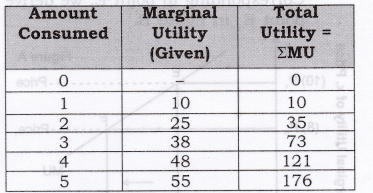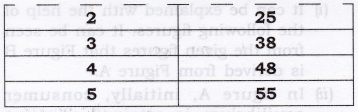As we know total utility is the sum total of marginal utilities as shown below.

Question 8. Calculate: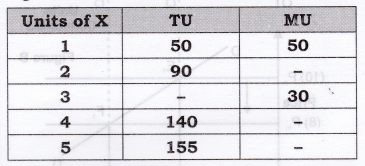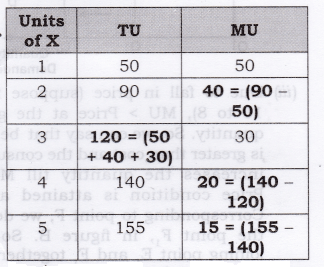Question 9. Derive the inverse relation between price of the good and its demand from single commodity equilibrium condition “marginal utility = price”. [CBSE Foreign 2011]
Answer: As we know a consumer purchases a good up to the point where marginal utility of the good becomes equal to the price of that good.
MU = Price

1. Figure B is derived from Figure A.
2. In figure A, initially, consumer equilibrium is attained at point E, where let MU (10) = Price (10). Corresponding to point E, we derive point E1 in figure B.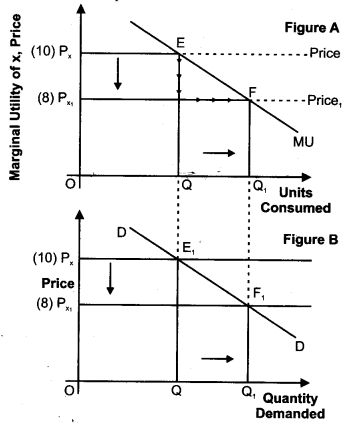3. Due to fall in price (suppose from 10 to 8), MU > Price at the given quantity. So, we can say that benefit is greater than cost and the consumer increases the quantity till MU = Price condition is attained at F. Corresponding to point F, we derive the point F1; in figure B. So, by joining point E1 and F1 together, we derive the demand curve.

Question 10.  A consumer consumes only two goods X and Y. At a consumption level of these two goods, he finds that the ratio of marginal utility to price in case of X is higher than that in case of Y. Explain the reaction of the consumer.  [AI 2011]
Or
A consumer consumes only two goods X and Y and is in equilibrium.
Price of X falls. Explain the reaction of the consumer through the Utility Analysis. Or  [AI 2012]
A consumer consumes only two goods X and Y and is in equilibrium. Price of good X falls. Show that it will lead to rise in demand for good X. Or  [CBSE 2013C]
By spending his entire income only on two goods X and Y a consumer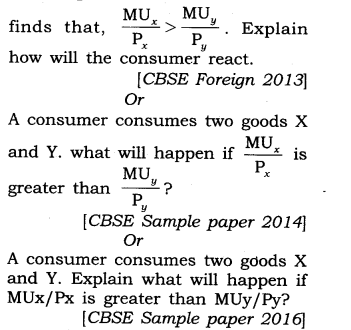Answer:  As, we know condition for consumer equilibrium is,
Necessary Condition
Marginal utility of last rupee spent on each commodity is same.
Suppose there are two commodities, X and Y respectively.
So, for commodity X, the condition is, Marginal Utility of Money = Price of X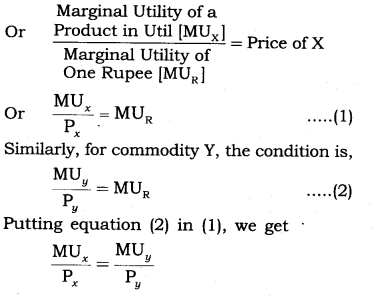But as given in the question that the ratio of marginal utility to price in case of X is higher than that in case of Y,i.e….
$$\frac { M{ U }_{ x } }{ { p }_{ x } } >\quad \frac { M{ U }_{ y } }{ { p }_{ y } }$$
It means marginal utility from the last rupee spent on commodity X is more than marginal utility from the last rupee spent on commodity Y. So, to attain the equilibrium consumer must increase the quantity of X, which decreases the MUx and decreases the quantity of Y, which will increase the MUy. Increase in quantity of X and decrease in quantity of Y till
$$\frac { M{ U }_{ x } }{ { p }_{ x } } =\quad \frac { M{ U }_{ y } }{ { p }_{ y } }$$

Question 11. A consumer consumes only two goods X and Y. At a certain consumption level of these goods, he finds that the ratio of marginal utility to price in case of X is lower than that in case of Y. Explain the reaction of the consumer. Or  [AI2011]
By spending his entire income only on two goods X and Y a consumer finds that,$$\frac { M{ U }_{ x } }{ { p }_{ x } } <\quad \frac { M{ U }_{ y } }{ { p }_{ y } }$$ Explain how will the consumed react.
Or
[CBSE Foreign 2013]
A consumer consumes only two goods X and Y and is in equilibrium. Show that when the price of good X rises, the consumer buys less of good X. Use utility analysis. [AI 2014]
Answer:  As, we know condition for consumer equilibrium is, Necessary Condition
Marginal utility of last rupee spend on each commodity is same.
Suppose there are two commodities, X and Y respectively.
So, for commodity X, the condition is, Marginal Utility of Money = Price of X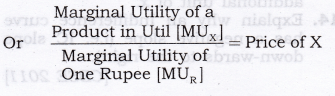But as given in the question that the ratio of marginal utility to price in case of X is lower than that in case of Y, i.e.,$$\frac { M{ U }_{ x } }{ { p }_{ x } } <\quad \frac { M{ U }_{ y } }{ { p }_{ y } }$$
It means, marginal utility from the last rupee spent on commodity X is less than the marginal utility from the last rupee spent on commodity Y. So, to attain the equilibrium the consumer must decrease the quantity of X which will increase the MUx and increase the quantity of Y, which will decrease the MU . Decrease in quantity of X and increase in quantity of Y continue till
$$\frac { M{ U }_{ x } }{ { p }_{ x } } =\quad \frac { M{ U }_{ y } }{ { p }_{ y } }$$

Question 12. Explain the meaning of diminishing marginal rate of substitution with the help of a numerical example. [AI 2013]
Answer: MRS is the rate at which a consumer is willing to sacrifice one commodity for an extra unit of another commodity without affecting his total satisfaction.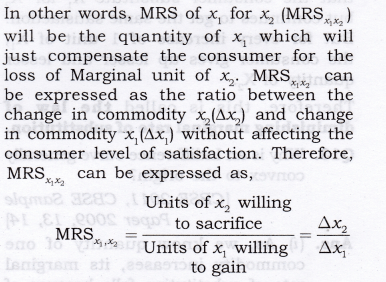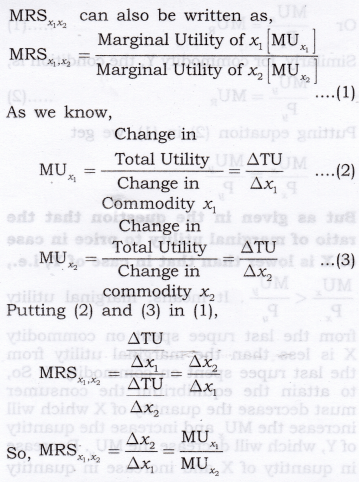It can be seen from the above schedule that the consumer substitute X1 for X2 but continues to get the same satisfaction. But for every increase of 1 unit of X1; the consumer gives up lesser and lesser quantity of X2.
Therefore, this is called the law of diminishing marginal rate of substitution.

Question 13. Why is an Indifference curve generally , convex to the origin? [CBSE 2011, CBSE Sample Paper 2009, 13, 14]

1. As, we know quantity of one commodity increases, its marginal rate of substitution falls because of law of diminishing marginal utility. Marginal rate of substitution is a slope of Indifference curve and whenever slope [MRS] decreases it makes the curve convex to the point of origin.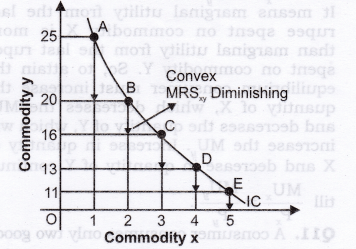2.  In the above diagram, units of y are measured on vertical axis and units of x on horizontal axis. When the consumer moves from combination A (1 x + 25y) to B (2x + 20y), he acquires one additional unit of x and forgoes (sacrifice) 5 units of y, if he wants to get the same level of satisfaction. The consumer has to reduce the consumption of y when he increases the consumption of x. The number of units of good y that the consumer is willing to sacrifice for an additional unit of good x, so as to maintain the same level of satisfaction is technically called the marginal rate of substitution of x for y and is denoted by MRSxy .
3. So, the MRSxy when the consumer move from combination A to B is 5 : 1, further as the consumer move from combination B to C, he acquires one more units of x, but the consumer forgoes a smaller number of y, i.e., MRSxy at this stage is 4 : 1. It may be observed now that MRS diminishes as the consumer moves from combination A to B, B to C, C to D, D to E. The consumer forgoes less and less units of y as he acquires additional unit of x.

Question 14. Explain why an Indifference curve has a negative slope (i.e. IC slope down-wards to the right). [CBSE 2011]
Answer:  Every IC is based on the assumption that various combinations of two commodities gives equal satisfaction to a consumer. In order to remain at the same level of satisfaction, the consumer will have to reduce the consumption of one commodity if he wants to increase the consumption of another commodity.Question 15. Why do Indifference curves not intersect each other?

1. Two IC’s cannot intersect each other. This property is proved by Contradict Method. First we assume that they intersect each other and then show that this assumption leads to an absurd conclusion. Let us assume that IC1 intersects IC2 at point E shown in the figure given here.2. Let point A be a point on IC, and point B on IC2. Since A and E lie on IC,, the consumer will be indifferent between points E and A (A = E). Similarly, B and E lie on IC2, the consumer will be indifferent between points E and B (B = E).
3.  Based on the assumption of transitivity as A = E and B = E, then the consumer must be indifferent between A and B (A = B) but this is not possible as A and B lie on two different ICs and represent different levels of satisfaction. Therefore, IC cannot intersect each other.

Question 16. Explain that Higher IC provides higher level of satisfaction, or
‘Higher indifference curve represents higher level of satisfaction to the consumer’. Explain the statement, also state the underlying assumption related to this property of indifference curve. [CBSE Sample Paper 2016]

1. Higher IC lying above and to the right of another IC represents a higher level of satisfaction. All combinations of goods X and Y lying on the higher indifference curve IC2 have more satisfaction than lower indifference curve IC1 as shown in figure given here.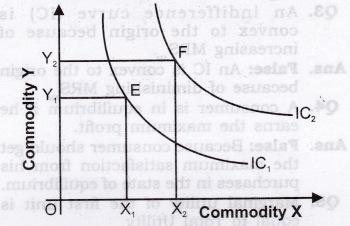2.  This is because of monotonic preferences, as monotonic preferences state that we must have atleast more of one good and no less that of other goods (means other goods can be equal or greater, but not less). The figure above shows that bundle F(OX2 + OY2) is monotonic preferred to bundle E(OX1 + OY1). So, if bundle F is monotonic preferred to bundle E than F bundle gives more satisfaction than that of Bundle E.
3. It can be seen from the above diagram that all combinations of IC2 contain a large quantity of both X and Y, than all combinations on IC,. For, e.g., point E lying on IC, represents OX, units of X and OY1 units of Y. Point F lying on IC2 represents more units of Y, i.e., OY, as well as more units of X, i.e. OX2.
The consumer gets greater satisfaction from a larger pieces of goods than from a smaller amount. Hence, point F shall be on a higher IC and shall be more preferable to point E, lying on lower IC.

IV.True Or False
Giving reasons, state whether the following statements are true or false.
Question 1. Total utility increases as long as marginal utility is positive (+).
Answer: True: Because TU is the sum of marginal utilities and MU is positive.

Question 2. TU starts declining when MU starts declining.
Answer: False: Because TU starts declining only when diminishing MU becomes negative.

Question 3. An indifference curve (IC) is convex to the origin because of increasing MRSxy.
Answer: False: An IC is convex to the origin because of diminishing MRS .

Question 4. A consumer is in equilibrium if he earns the maximum profit.
Answer: False: Because consumer should get the maximum satisfaction from his purchases in the state of equilibrium.

Question 5. Marginal utility of the first unit is equal to Total Utility.
Answer: True: MU = TUn – TUn-1 = TU1 – TU0= TU1

Question 6. In case of single commodity, consumer will be in equilibrium when M.U. = Income.
Answer: False: A consumer is in equilibrium when MU = Price.

Question 7.  If there are two commodities, consumer is in equilibrium if $$\frac { M{ U }_{ x } }{ Income } =\frac { M{ U }_{ y } }{ Income }$$.
Answer: False: A consumer is in equilibrium only when $$\frac { M{ U }_{ x } }{ { P }_{ x } } =\frac { M{ U }_{ y } }{ { P }_{ y } }$$.Because here consumer1 behaviour matches with the market behaviour.

Question 8. If TU remains the same; MU may be negative or positive.
Answer:  False: TU decreases if MU is negative, ‘ and TU increases if MU is positive.
It is constant and maximum only if MU = 0.

Question 9. In case of indifference curve consumer is in equilibrium if $$MR{ S }_{ xy }=\frac { { P }_{ x } }{ { P }_{ y } }$$.
Answer: True: Here consumer’s behaviour and market behaviour match with each other.

Question 10. A consumer is in equilibrium where indifference curve equals budget line?
Answer:  False: Consumer will be in equilibrium where IC is tangent to the budget line.

Question 11.  All points on the budget line give equal satisfaction to the consumer.
Answer:  False: All points on an IC give equal satisfaction.

Question 12. All combinations on an IC are achievable by a consumer.
Answer: False: All the combinations on a budget line are achievable and not points on IC.
Note: As per CBSE guidelines, no marks will be given if reason to the answer is not explained.

V. Long Answer Type Questions (6 Marks)
Question 1. Explain consumer’s equilibrium in case of a single commodity with the help of a utility schedule. [CBSE 2005, 06, 07C, 09, AI 05, 08,Foreign 2009] Or
State condition of consumer equilibrium in case of a single commodity. Or [CBSE 2006]
There is given the market price of a piece of goods, how does a consumer decides as to how many units of that piece of goods to buy.  [AI 2008, 09] Or
How many units of a commodity should a consumer buy to get its maximum utility? Explain with the help of a numerical example. [Foreign 2005]
Or
There is given the price of a good, how does a consumer decide as to how much quantity of that goods to buy? [CBSE 2012)

1. When purchasing a unit of a commodity a consumer compares its price with the expected utility from it. Utility obtained is the benefit, and the price payable is the cost. The consumer compares benefit and the cost. He will buy the unit of a commodity only if the benefit is greater than or at least equal to the cost.
2. Equilibrium Conditions for Single Commodity Consumer Equilibrium.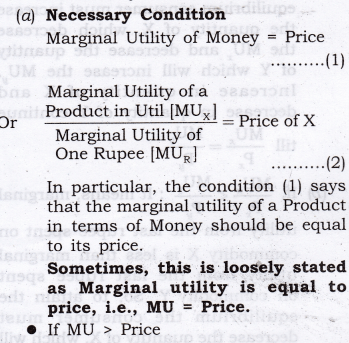> As a rational consumer he will continue to purchase an additional unit of a commodity as long as MU = Price.
> MU > Price implies benefit is greater than cost and whenever benefit is greater than cost, the consumer keeps on consuming additional unit of a commodity till MU = Price.
> It is so because according to the law of diminishing marginal utility MU falls as more is purchased. As MU falls it is bound to become equal to the price at some point of purchase.
• If MU< Price
> As a rational consumer he will have to reduce the consumption of a commodity as long as MU = Price.
> MU < Price implies when benefit is less than cost, and whenever benefit is less than cost the consumer keeps on decreasing the additional unit of a commodity till MU = Price.
> It is so because according to the law of diminishing marginal utility, MU rises as less units are consumed. As MU rises, it is bound to become equal to the price at some point of purchase.
(b) Sufficient Condition: Total gain falls as more is purchased after equilibrium. It means that consumer continues to purchase so long as total gain is increasing or at least constant.
3.  It can be explained with the help of the following schedule:(a) Suppose, the price of commodity X in the market is Rs3 per unit. It means he has to pay ?3 per unit for all the units he buys. Suppose, the utility obtained from the first unit is 5 utils (= Rs5). The consumer will buy this unit because the utility of this unit is greater than the price and this process continues till Marginal utility = Price as shown in the above schedule at quantity 3.
(b) Consumer will not buy the fourth unit because utility of this unit is 2 units (= Rs2) which is less than the price. It is not worth buying the fourth unit. The consumer will restrict his purchase to only 3 units.

Question 2. A consumer consumes only two goods. Explain the conditions of the consumer’s equilibrium with the help of Utility Analysis.
[CBSE Sample Paper 2013] Or
State and explain the condition of consumer’s equilibrium in case of two commodities through utility approach.[CBSE 2009C, Foreign 2005, 2010]
Answer:  According to the two commodity consumer equilibrium or law of Equimarginal utility, a consumer gets maximum satisfaction, when ratios of MU of two commodities and their respective prices are equal. Conditions of Consumer’s Equilibrium in case of two commodities

1. Necessary Condition
Marginal utility of last rupee spend on each commodity is same. Suppose there are two commodities, X and Y respectively.
So, for commodity X, the condition is, Marginal Utility of Money = Price of X(a) If $$\frac { M{ U }_{ x } }{ { P }_{ x } } >\frac { M{ U }_{ y } }{ { P }_{ y } }$$ : It means, marginal utility from the last rupee spent on commodity X is more than marginal utility from the last rupee spent on commodity Y. So, to attain the equilibrium consumer must increase the quantity of X, which decrease the MUx and decrease the quantity of Y which will increase the MUy .Increase in quantity of X and decrease in quantity of Y continue till $$\frac { M{ U }_{ x } }{ { P }_{ x } } =\frac { M{ U }_{ y } }{ { P }_{ y } }$$
(b) If,$$\frac { M{ U }_{ x } }{ { P }_{ x } } <\frac { M{ U }_{ y } }{ { P }_{ y } }$$
It means, marginal utility from the last rupee spent on commodity X is less than marginal utility from the last rupee spent on commodity Y. So, to attain the equilibrium the consumer must decrease the quantity of X, which will increase the MUx and increase the quantity of Y, which will decrease the MUy. Decrease in quantity of X and increase in quantity of Y continues till $$\frac { M{ U }_{ x } }{ { P }_{ x } } =\frac { M{ U }_{ y } }{ { P }_{ y } }$$
2. Sufficient Condition
Expenditure on commodity X + Expenditure on commodity Y = Money Income. In other words, Marginal utility falls as more units of a commodity are consumed. This condition must be satisfied to attain the necessary condition, i.e.,$$\frac { M{ U }_{ x } }{ { P }_{ x } } =\frac { M{ U }_{ y } }{ { P }_{ y } }$$.If MU does not fail as consumption of a commodity increases, the consumer will spend all his income on one commodity, which is highly unrealistic.

Question 3. For a consumer to be in equilibrium why must marginal rate of substitution be equal to the ratio of prices of the two goods? [CBSE Sample Paper 2009] Or
Using indifference curve approach, explain the conditions of consumer’s equilibrium. [CBSE 2010, IOC, 11C, Foreign 2010, AI 11]
Or
Why is the consumer in equilibrium when he buys only that combination of the two goods that is shown at the point of tangency of the budget line with an indifference curve? Explain. [CBSE Sample Paper 2009] Or
What are the conditions of consumer’s equilibrium under the indifference curve approach? What changes will take place if the conditions are not fulfilled to reach equilibrium? Or  [Al 2010]
State and explain the conditions of consumer’s equilibrium in indifference curve analysis. [CBSE 2013, C]
Or
Explain consumer equilibrium using the concept of budget line and indifference map or Interior Optimum Consumer Equilibrium.
Or
A consumer consumes only two of goods. For the consumer to be in equilibrium why must Marginal Rate of Substitution between the two goods must be equal to the ratio of prices of these two goods? Is it enough to ensure equilibrium?
[CBSE Sample Paper 2013] Or
A consumer consumes only two goods. Explain the conditions that need to be satisfied for the consumer to be in equilibrium under indifference curve analysis. [CBSE Sample Paper 2014] Or
Show diagrammatically the conditions for consumer’s equilibrium, in Hicksian analysis of demand.  [CBSE Sample Paper 2016]
Answer: (i) To define consumer equilibrium, we use Indifference Curve map and the budget line.
Two Conditions for Consumer Equilibrium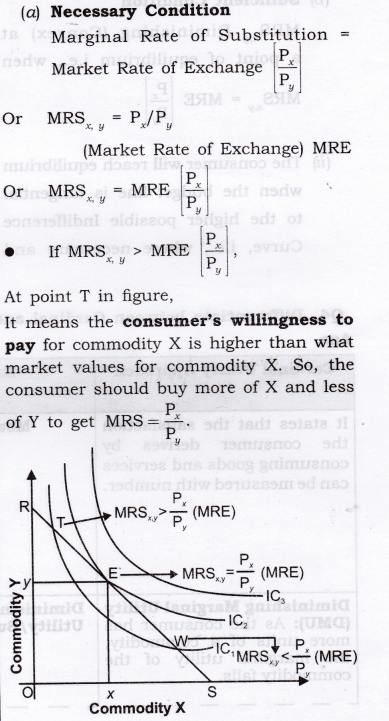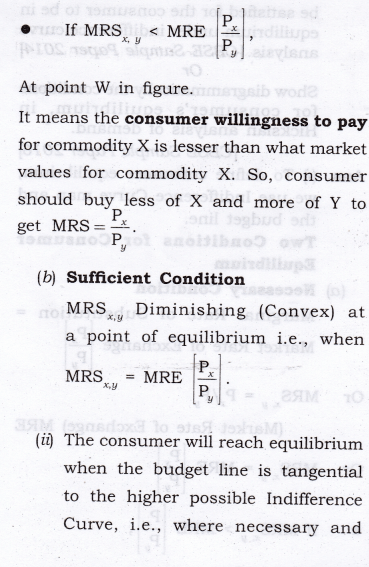sufficient conditions satisfy. In the above diagram, the consumer will reach equilibrium at point E where budget line RS is tangential to the highest possible IC2.
(iii) The consumer cannot move to Indifference Curve, i.e., IC3 as this is beyond his money income.
(iv) Even on IC2, all the other points except E are beyond his means.
(v) Hence, at point E, the consumer is in equilibrium where his satisfaction maximizes, given his income and prices of goods X and Y. In equilibrium at E, the slope of Budget line = the slope of Indifference Curve. Therefore, MRSxy is equal to the ratio of the prices of two goods
$$\left\lceil \frac { { P }_{ x } }{ { P }_{ y } } \right\rceil$$

Question 4. Differentiate between Cardinal and Ordinal Utility. [AI 2012]VI. Higher Order Thinking Skills (Hots)
Question 1. What does budget line in terms of price and money income indicate? [1 Mark]
Answer:  Budget line in terms of prices and money income indicate that prices are implicitly in the budget line.

Question 2. What is the slope of budget line? [1 Mark]
Answer: The slope of budget line
$$=\frac { Vertical(Y-axis)intercept }{ Horizontal(X-axis)intercept } =\frac { \frac { M }{ { P }_{ 2 } } }{ \frac { M }{ { P }_{ 1 } } } =\frac { { P }_{ 1 } }{ { P }_{ 2 } }$$

Question 3. What is the horizontal (X-axis) intercept of budget line? [J Mark]
Answer: Horizontal intercept of budget line
$$=\frac { Money\quad income }{ Price\quad of\quad the\quad commodity\quad on\quad horizontal\quad axis } =\frac { { M } }{ { P }_{ 1 } }$$

Question 4. What is the Vertical (Y-axis) intercept of budget line?
Answer. Vertical intercept of budget line
$$=\frac { Money\quad income }{ Price\quad of\quad the\quad commodity\quad on\quad vertical\quad axis } =\frac { { M } }{ { P }_{ 2 } }$$

Question 5. “Law of diminishing marginal utility will operate even if consumption takes place in intervals.” Defend or refute. [3-4 Marks]
Answer: The given statement is refuted. Law of diminishing marginal utility will operate only when consumption is a continuous process. For example, if one sandwich is consumed in the morning and another in the afternoon, the second sandwich may provide equal or higher satisfaction as compared to the first one.

Question 6. Derive the law of demand from the two commodity equilibrium condition “Marginal Utility = price ratio through utility approach”.  [3-4 Marks]

1. The law states that a consumer is in equilibrium when the ratio of MU to price in case of each good consumed is the same. In of goods, X and Y, a consumer is in equilibrium when,$$\frac { M{ U }_{ x } }{ { P }_{ x } } =\frac { M{ U }_{ y } }{ { P }_{ y } }$$.
2. Given that the consumer is in equilibrium and price of X falls. It can be seen from the figures that Figure B is derived from Figure A.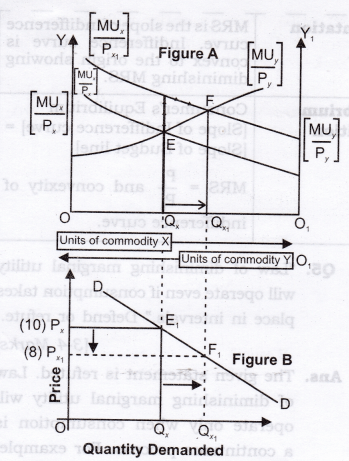3.  In figure A, initially, the consumer equilibrium is attained at point E,where $$\frac { M{ U }_{ x } }{ { P }_{ x } } =\frac { M{ U }_{ y } }{ { P }_{ y } }$$ (Assuming, Px =10). Corresponding to point E, we derive point E1 in figure B.
4. Due to fall in price (suppose from 10 to 8), $$\frac { M{ U }_{ x } }{ { P }_{ x } } >\frac { M{ U }_{ y } }{ { P }_{ y } }$$ ,at the given quantity Qx. It means, marginal utility from the last rupee spent on commodity X is more than marginal utility from the last rupee spent on commodity Y. So, to attain the equilibrium the consumer must increase the quantity of X, which decreases the MUx and decreases the quantity of Y, which will increase the MUy. Increase in quantity of X and decrease in quantity of Y continue $$\frac { M{ U }_{ x } }{ { P }_{ x } } =\frac { M{ U }_{ y } }{ { P }_{ y } }$$ and the new consumer equilibrium will be attained at point F. Corresponding to point F, we derive the point F1, in figure B. So, by joining point E1 and F1, we derive the demand curve.

Question 7. Define market rate of exchange. [3-4 Marks]
Answer: The rate at which market requires to sacrifice one commodity to gain an additional unit of another commodity is called market rate of exchange.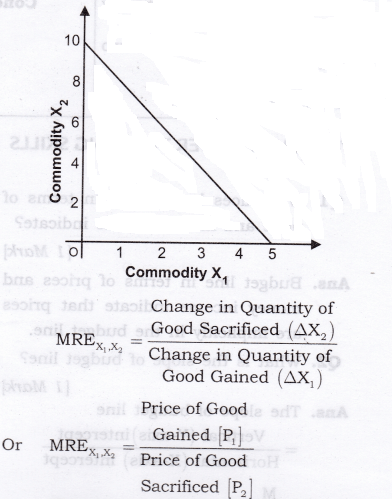VII. Value Based Questions
Question 1. How is the law of diminishing marginal utility applied with regard to education/ knowledge? [1 Mark]
Answer: In this case the law of diminishing marginal utility will not apply because every effort to get education/ knowledge increases the utility. Value: Analytic

VIII. Application Based Questions
Question 1. A consumer has Rs 10, and both goods X and Y are priced at Rs 2 and are available in integer units, (a) give the bundles that this consumer can afford to buy (bj give the bundles that cost exactly Rs 10 (c) give two bundles that this consumer cannot afford to buy. [3-4 Marks]
(0,0), (0,1), (0,2), (0,3), (0,4), (0,5),
(1.0), (1,1), (1,2), (1,3), (1,4), (2,0),
(2.1), (2,2), (2,3), (3,0), (3,1), (3,2), (4,0), (4,1) and (5,0).
(b) Bundles that cost exactly ?10 are, (0,5), (1,4), (2,3), (3,2), (4,1), (5,0).
(c) Two bundles that the consumer cannot afford to buy are, (3, 3), (4, 2).

Question 2. A consumer has Rs 40 and both goods X and Y are priced at Rs 20 and are available in integer units, (a) Give the bundles that this consumer can afford (b) give the bundles that cost exactly Rs 40. [3-4 Marks]
Answer: (a) Affordable bundles are, (0,0), (0,1),(0,2),(1,0),(1,1),(2,0).
(b) Bundles that cost exactly Rs 40 are, (0, 2), (1, 1), (2, 0).

Question 3. Give reasons for the following statements:  [3-4 Marks]

1.  If the income of a consumer changes and prices of the two goods remain unchanged, a new budget line will be formed which will be parallel to the original line.
2. If the income of the consumer remains unchanged and if the price of goods X rises, intercept of the budget line of Y-axis will remain the same, but on the X-axis it will shift to the left.

1.  As we know, slope of budget line is Px (i.e. price ratio of two goods) with the increase in income. remains constant and only ability to purchase quantities of both commodity X and commodity Y increases. Hence, the budget line shift parallel to the right because when slope is same, than curve should be parallel.
2. In this case, the price ratio between X and Y changes. Since the price of X rises, the consumer is in a position to purchase lesser quantity of X while his ability to purchase Y remains unchanged.

Question 4. Giving reasons, comment on the following statements:  [3-4 Marks]

1. A consumer’s equilibrium is always formed at a point on the given budget line.
2.  A consumer’s equilibrium will shift to a higher indifference curve with an increase in consumer’s income.